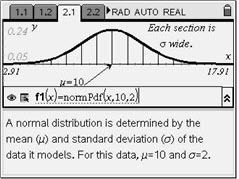# Activities

••• ##### Subject Area

• Math: Algebra II: Data Analysis

• ##### Author9-12

45 Minutes

• ##### Device
• TI-Nspire™
• TI-Nspire™ CAS
• ##### Software

TI-Nspire™
TI-Nspire™ CAS

1.7

• ##### Other Materials
By Lynne Plettenberg

## Average Orange#### Activity Overview

This activity presents the normal probability distribution, beginning with the normal curve. Students explore the area under the curve between various x-values and use a model to find what percent of the area lies within 1, 2, and 3 standard deviations of the mean. They then apply this knowledge to answer questions about an orange crop with normally distributed weights. Percentiles are defined and calculated.

#### Key Steps

•The shape of a normal curve is controlled by two parameters: the mean, which controls the location of the hump of the curve, and the standard deviation, which controls whether the bell is broad and flat (larger standard deviation) or narrow and tall (smaller standard deviation). If the bell curve represents the distribution of some measurement across a population, the mean is the population average and the standard deviation measures the spread of the population measurements.